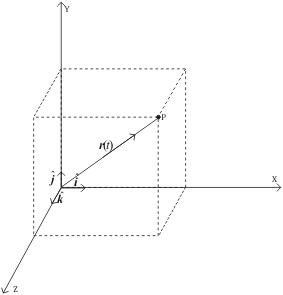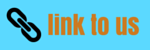# Motion in three dimensions

## 8. Motion in three dimensions

• We have already studied physical quantities like displacement ,velocity,acceleration etc in one and two dimension
• In this topic ,we will generalize our previous knowledge of motion in 1 and 2 -dimension to three dimension's
• As we have used vectors to represent motion in a plane,we can freely use vectors and its properties in 3-dimension as we have done in case of motion in a plane
• In three dimensions ,we have three units vectors i ,j and k associated with each co-ordinate axis of Cartesian co-ordinates system shown below in the figure• Consider a particle moving in 3-D space .Let P be its position at any point t.Position vector of this particle at point P would be
r=xi+yj+zk
Where x,y and z are co-ordinates of point P
• Similarly velocity and acceleration vectors of particle moving in 3-D space are
v=vxi+vyj+vzk where vx=dx/dt,vy=dy/dt and vz=dz/dt
and
a=vxi+ayj+azk
where ax=dvx/dt,ay=dvy/dt and az=dvz/dt
• All the relations we have derived in case of motion in plane are valid for 3-D motion with one added co-ordinate